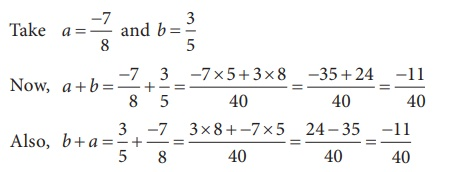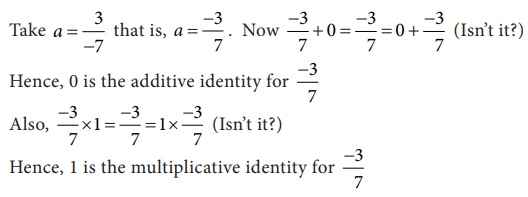Home | | Maths 8th Std | Properties of Rational Numbers

# Properties of Rational Numbers

Some properties listed here below will be of good use in solving problems.

Properties of Rational Numbers

Some properties listed here below will be of good use in solving problems.

1. Closure property/law for the collection of rational numbers

For any two rational numbers a and b, the sum a + b is also a rational number.

ii) Closure property for Multiplication

For any two rational numbers a and b, the product ab is also a rational number.

IllustrationTry this

The closure property on integers holds for subtraction and not for division. What about rational numbers? Verify.

Solution:

Let 0 and 1/2 be two rational numbers 0 – 1/2 is a rational number

Closure property for subtraction holds for rational numbers.

But consider the two rational number 5/2 and 0.

5/2  ÷ 0 = 5 / [2 × 0] = 5 / 0

Here denominator = 0 and it is not a rational number.

Closure property is not true for division of rational numbers.

2. Commutative property/law for the collection of rational numbers

For any two rational numbers a and b, a + b = b + a.

ii) Commutative property for Multiplication

For any two rational numbers a and b, ab = ba (ab means a × b and ba means b × a).

IllustrationHere, we find that a + b = b + a and hence addition is commutative.

Further,Here, we find that a × b = b × a and hence multiplication is commutativeSolution:

Is 3/5 – 7/8 = 7/8 – 3/5 ?LHS = 3/5 + 7/8 = [ (3 × 8) – (7 × 5) ] / 40 = [24 – 35] / 40 = −11/40

RHS = 7/8 − 3/5 = [ (7 × 5) – (3 × 8) ] / 40 = [35 – 24] / 40 = 11/40

LHS  ≠  RHS

3/5  ÷ 7/8  ≠ 7/8 – 3/5

Subtraction of rational numbers is not commutative.

(ii) Is 3/5 ÷ 7/8 = 7/8 – 5/3 ?LHS = 3/5  ÷  7/8 = 3/5  × 8/7 = 24/35

RHS = 7/8  ÷  5/3 = 7/8  × 3/5 = 21/40

LHS  ≠  RHS

3/5  ÷ 7/8  ≠ 7/8 ÷ 5/3

Commutative property not hold good for division of rational numbers.3. Associative property/law for the collection of rational numbers

For any three rational numbers a, b, and c, a + (b + c) = (a + b) + c

ii) Associative property for Multiplication

For any three rational numbers a, b, and c, a(bc) = (ab)c

Illustration

Take rational numbers a , b, c as a = 1/2 , b = 3/5 and c = 7/10(3) and (4) shows that

(a × b) × c = a × (b × c) is true for rational numbers. Thus, the associative property is true for addition and multiplication of rational numbers.

Try this

Check whether associative property holds for subtraction and division.

Solution:Consider the rational numbers 2/3, 1/2  and 3/4

To verify (2/3 – 1/2) – 3/4 = 2/3 – (1/2 – 3/4)

LHS = (2/3 – 1/2) – 3/4 = ( [(2 × 2) – (1 × 3)] / 6 ) – (3/4)

= ([4 – 3]/6) – 3/4 = 1/6 – 3/4 = [(1 × 2) – (3 × 3)] / 12 = [2 – 9] / 12 = – 7 / 12

RHS = 2/3 – (1/2 – 3/4) = 2/3 – ( [2 – 3] / 4) =  ( 2/3 −  (−1/4) )

= 2/3 + 1/4  = [ (2 × 4) + ( 1 × 3) ] / 12 = [ 8 + 3] / 12 = 11 / 12

LHS  ≠  RHS

∴ ( 2/3 – 1/2) – 3/4  ≠ 2/3  − (1/2 − 3/4)

Associative property not holds for subtraction of rational numbers

Also to verify (2/3 ÷ 1/2) ÷ 3/4 = 2/3 ÷ (1/2 ÷ 3/4)

LHS = (2/3  ÷  1/2) ÷  3/4 = (2/3 × 2/1) ÷ 3/4

= 4/3  ÷ 3/4 = 4/3 × 4/3 = 16 / 9

RHS = 2/3 ÷ (1/2 ÷ 3/4) = 2/3 ÷ ( 1/2 × 4/3) =  2/3 ÷  ( 2/3 )

= 2/3 × 3/2 = 1

LHS  ≠  RHS

ie. (2/3 ÷ 1/2) ÷ 3/4 ≠ 2/3 ÷ ( 1/2 ÷ 3/4)

Associative property does not hold for division of rational numbers.

4. Identity property/law for the collection of rational numbers

For any rational number a, there exists a unique rational number 0 such that

0 + a = a = 0 + a.

ii) Identity property for Multiplication

For any rational number a, there exists a unique rational number 1 such that

1 × a = a = a × 1.

IllustrationTake a = 3/-7 that is, a = -3/7 . Now -3/7 + 0 = -3/7 = 0 + -3/7 (Isn’t it?)

Hence, 0 is the additive identity for −3/7

Also, -3/7 × 1 = - 3/7 = 1 × -3/7 (Isn’t it?)

Hence, 1 is the multiplicative identity for −3/7

5. Inverse property/law for the collection of rational numbers

For any rational number a, there exists a unique rational number a such that a + (a) = (a) + a = 0. Here, 0 is the additive identity.

ii) Multiplicative Inverse property

For any rational number b, there exists a unique rational number 1/b such that b × 1/ b = 1/b × b = 1 . Here, 1 is the multiplicative identity.

Illustration6. Distributive property/law for the collection of rational numbers

Multiplication is distributive over addition for the collection of rational numbers. For any three rational numbers a, b and c, the distributive law is a × (b +c) = ( a × b) +(a × c)

Illustration(1) and (2) shows that a × (b + c ) = (a × b) + (a × c) .

Hence, multiplication is distributive over addition for the collection of rational numbers.

Tags : Numbers | Chapter 1 | 8th Maths , 8th Maths : Chapter 1 : Numbers
Study Material, Lecturing Notes, Assignment, Reference, Wiki description explanation, brief detail
8th Maths : Chapter 1 : Numbers : Properties of Rational Numbers | Numbers | Chapter 1 | 8th Maths